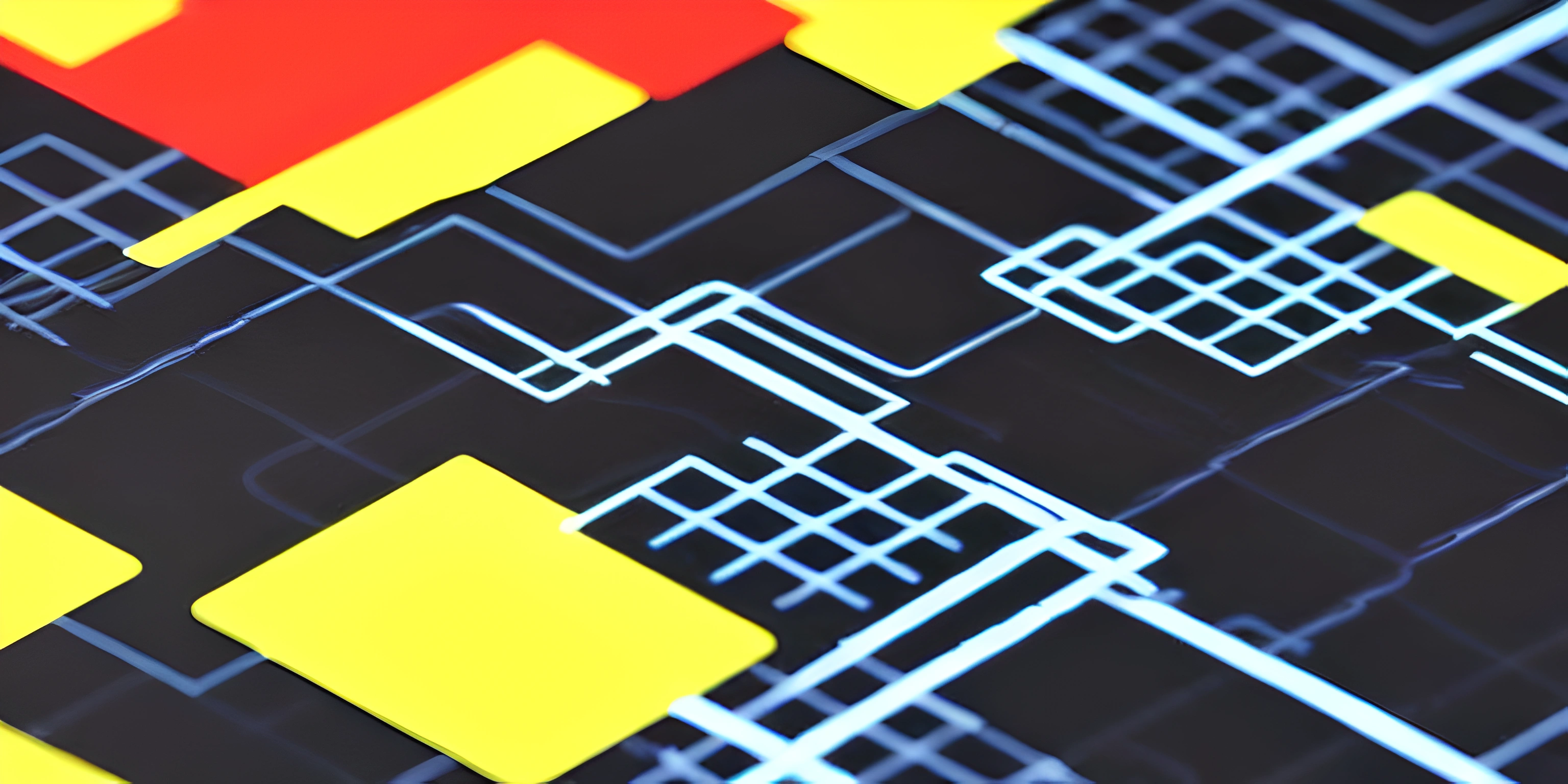# Understanding p5.Vectors and Their Role in p5.jsNote: this page has been created with the use of AI. Please take caution, and note that the content of this page does not necessarily reflect the opinion of Cratecode.

p5.js is a powerful library for creating graphics and animations in JavaScript. One of the key components of p5.js is the `p5.Vector` object, which represents a vector in two or three-dimensional space. In this article, we'll dive into p5.Vectors, exploring their properties and methods, and how they play an essential role in creating complex graphics and animations.

## What is a p5.Vector?

A `p5.Vector` is a mathematical object that represents a position, velocity, or acceleration in 2D or 3D space. Vectors are essential in p5.js because they allow us to perform arithmetic and manipulate coordinates more efficiently than using individual variables for each axis.

### Creating a p5.Vector

You can create a p5.Vector using the `createVector()` function provided by p5.js. This function takes in two or three arguments for the x, y, and z components of the vector. For example:

``````// Create a 2D vector with x = 3 and y = 4
let myVector = createVector(3, 4);

// Create a 3D vector with x = 1, y = 2, and z = 3
let my3DVector = createVector(1, 2, 3);``````

### Properties and Methods

p5.Vectors come with built-in properties and methods that enable you to manipulate and perform operations on them. Here are some examples:

``````let v1 = createVector(3, 4);
let v2 = createVector(1, 2);

v1.add(v2); // v1 becomes (4, 6)

// Subtract v2 from v1
v1.sub(v2); // v1 becomes (3, 4)

// Multiply v1 by a scalar
v1.mult(2); // v1 becomes (6, 8)

// Normalize v1 (make its magnitude 1)
v1.normalize(); // v1 becomes approximately (0.6, 0.8)

// Get the magnitude of v1
let magnitude = v1.mag(); // magnitude is 1``````

## Using p5.Vectors in Graphics and Animations

p5.Vectors are particularly useful when dealing with position, velocity, and acceleration in graphics and animations. They enable you to manipulate and update these quantities more efficiently, resulting in cleaner and more maintainable code.

### Example: Moving Ball with Vectors

Consider a simple animation of a moving ball. Using p5.Vectors for the ball's position and velocity simplifies the code and makes it easier to understand:

``````let ballPos, ballVel;

function setup() {
createCanvas(640, 480);
ballPos = createVector(width / 2, height / 2);
ballVel = createVector(2, 3);
}

function draw() {
background(255);

// Update the ball's position using the velocity vector

// Check for collisions with the canvas edges and reverse velocity if necessary
if (ballPos.x < 0 || ballPos.x > width) ballVel.x *= -1;
if (ballPos.y < 0 || ballPos.y > height) ballVel.y *= -1;

// Draw the ball
fill(0);
ellipse(ballPos.x, ballPos.y, 20, 20);
}``````

In this example, we use a position vector (ballPos) and a velocity vector (ballVel) to control the movement of the ball. The `add()` method makes it easy to update the position based on the velocity, and the code is more readable and maintainable.

In conclusion, understanding p5.Vectors and their role in p5.js is crucial for creating complex graphics and animations. Embracing the power of vectors will make your life as a p5.js programmer more comfortable and enable you to build exciting and creative projects.

## FAQ

### What is a p5.Vector in p5.js?

A p5.Vector is a special class in p5.js that represents a 2D or 3D vector. It's a convenient way to store and manipulate x, y, and z coordinates, making it easier to handle various calculations for graphics and animations. p5.Vectors are an essential part of p5.js programming.

### How do I create a new p5.Vector object?

To create a new p5.Vector object, you can use the `createVector()` function. This function accepts up to three arguments, representing the x, y, and z coordinates of the vector. If no arguments are passed, it initializes the vector with all coordinates set to 0. Here's an example:

``let myVector = createVector(5, 10, 2);``

### How can I perform basic vector operations like addition and subtraction?

p5.Vector provides several methods for common vector operations. For example, to add or subtract two vectors, you can use the `add()` and `sub()` methods, respectively. Here's an example:

``````let vectorA = createVector(3, 4);
let vectorB = createVector(1, 2);
// Subtract vectors
let difference = vectorA.sub(vectorB);``````

### Can I calculate the distance between two points using p5.Vector?

Yes, you can use the `dist()` method to calculate the distance between two points represented by p5.Vector objects. For example:

``````let pointA = createVector(1, 1);
let pointB = createVector(4, 5);
// Calculate the distance between pointA and pointB
let distance = pointA.dist(pointB);``````

### How do I normalize a vector in p5.js?

To normalize a vector (i.e., set its magnitude to 1), you can use the `normalize()` method. This is helpful when you want to work with a vector's direction, without considering its magnitude. Here's how you can normalize a vector:

``````let myVector = createVector(3, 4);
// Normalize the vector
myVector.normalize();``````